NCERT Exemplar Class 12 Maths Chapter 9 Differential Equations are part of NCERT Exemplar Class 12 Maths. Here we have given Exemplar Problems for Class 12 Maths Chapter 9 Differential Equations PDF.

## NCERT Exemplar Class 12 Maths Chapter 9 Differential Equations

NCERT Exemplar Class 12 Maths Chapter 9 Differential Equations Solutions is given below.2. Find the differential equation of all non-vertical lines in a plane.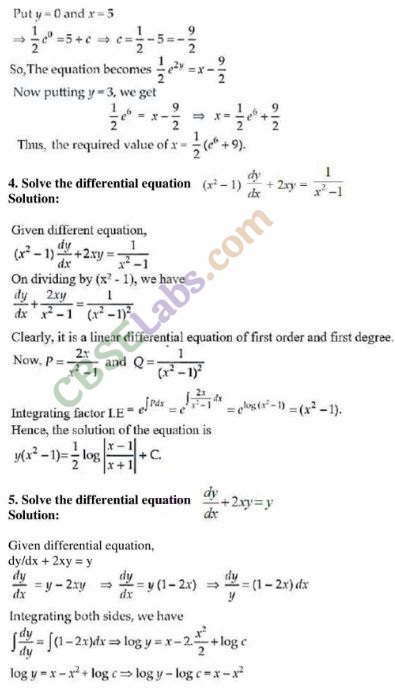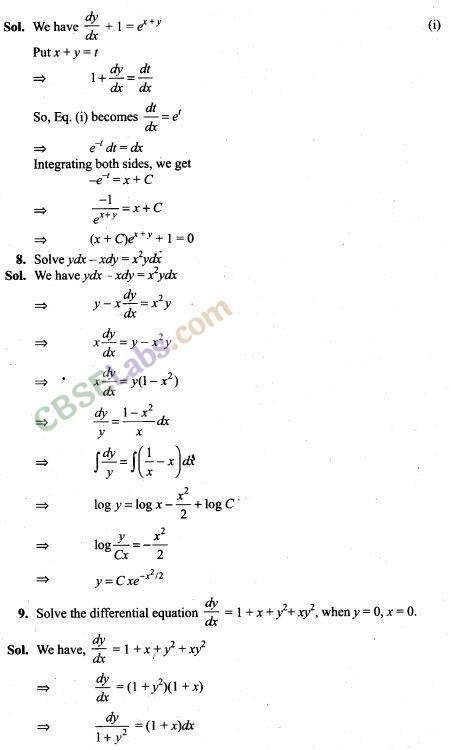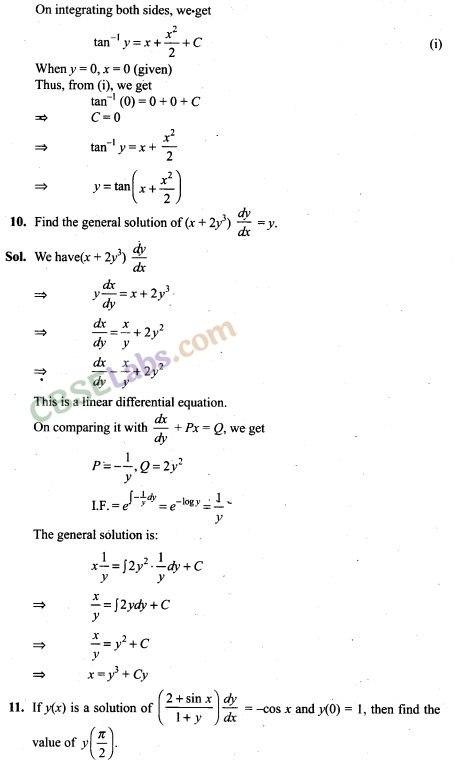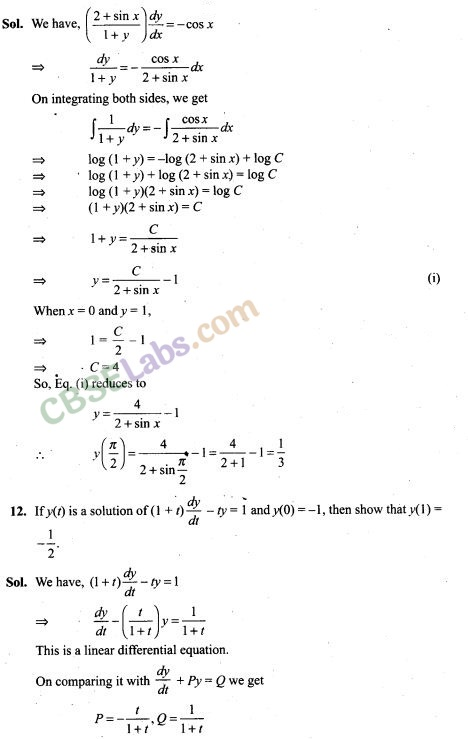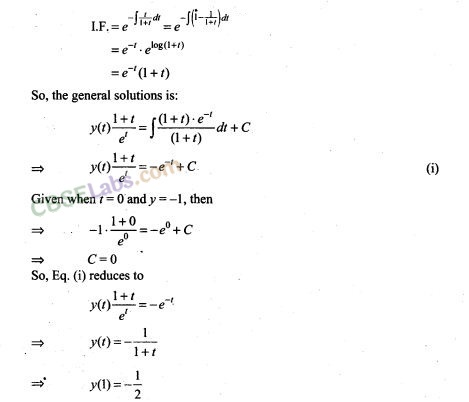13. Form the differential equation having y = (sin-1 x)2+ A cos-1 x + B, where A and B are arbitrary constants, as its general solution.14. Form the differential equation* of all circles which pass through origin and whose centres lie on y-axis.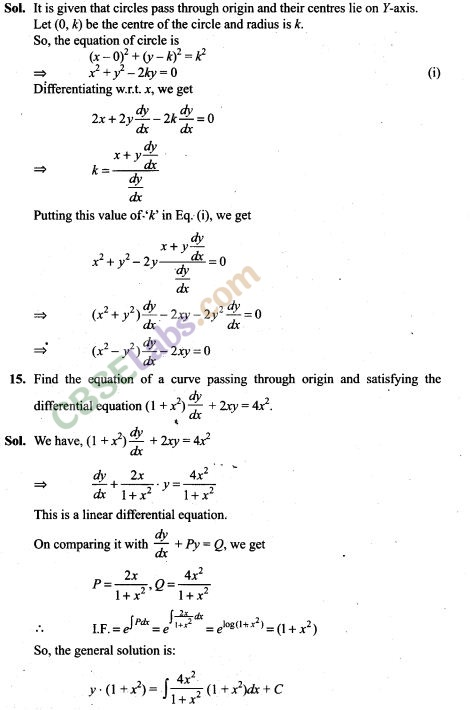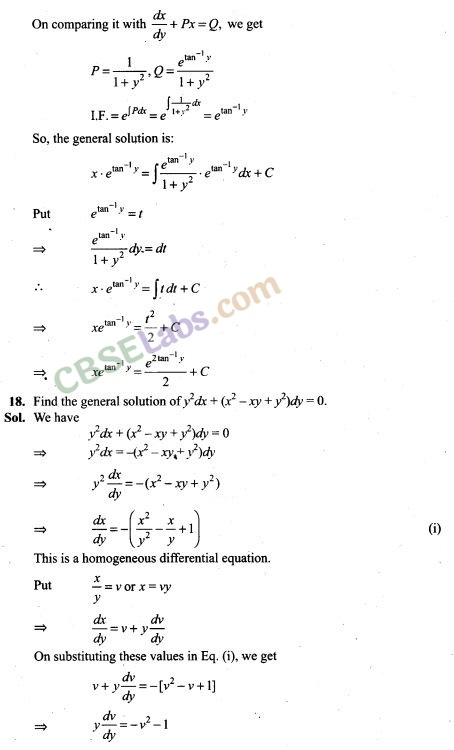19. Solve : (x + y)(dx -dy) = dx + dy
[Hint: Substitute x+y = z after separating dx and dy]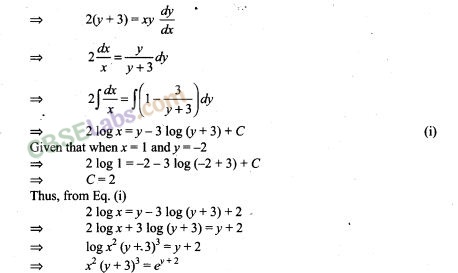21. Solve the differential equation dy = cos x (2 – y cosec x) dx given that y=2 when x = π /2.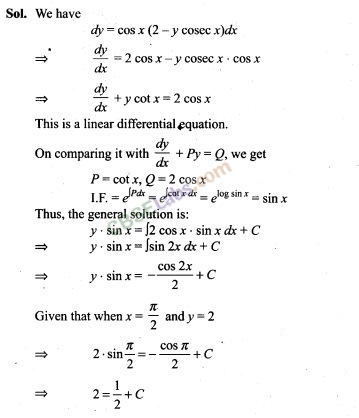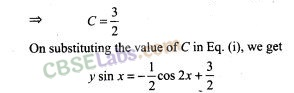22. Form the differential equation by eliminating A and B in Ax2 -By2 = 1.23. Solve the differential equation (1 +y2) tan-1 x dx + 2y (1+x2)dy=0.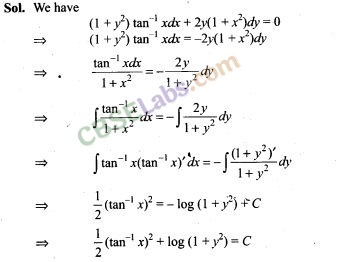24. Find the differential equation of system of concentric circles with centre (1,2).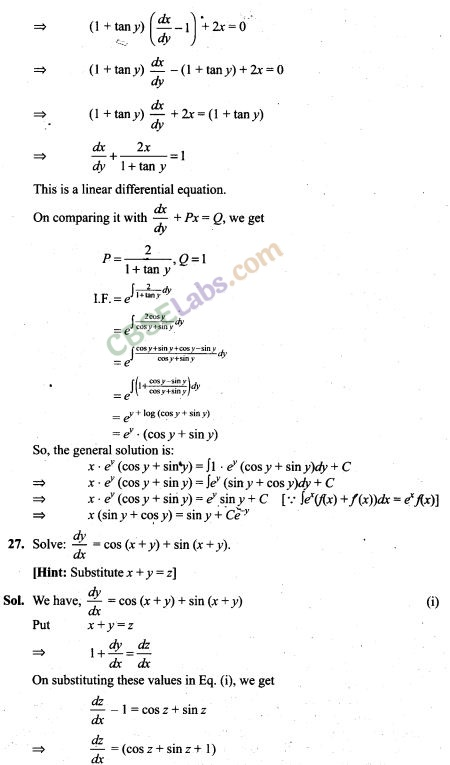31. Find the equation of a curve passing through origin if the slope of the tangent to the curve at any point (x, y) is equal to the square of the difference of the abscissa and ordinate of the point.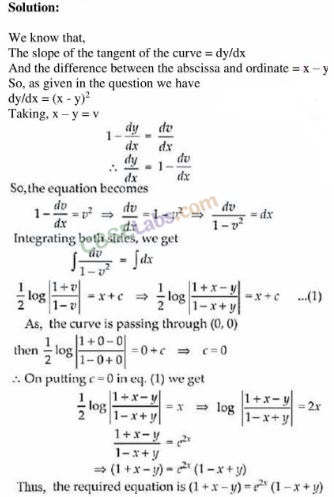32. Find the equation of a curve passing through the point (1, 1). If the tangent drawn at any point P(x, y) on the curve meets the co-ordinate axes at A and B such that P is the mid-point of AB.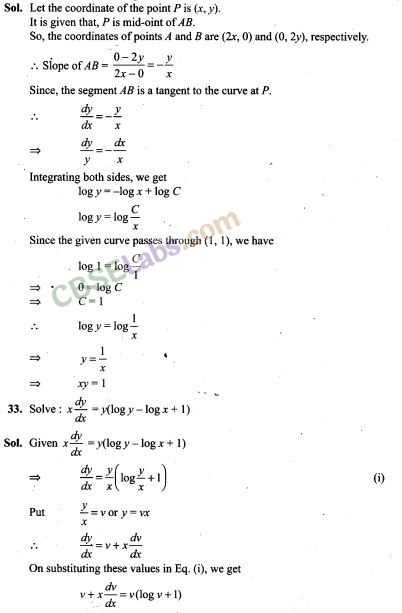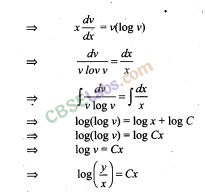Objective Type Questions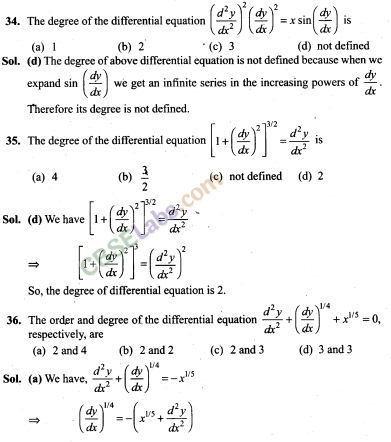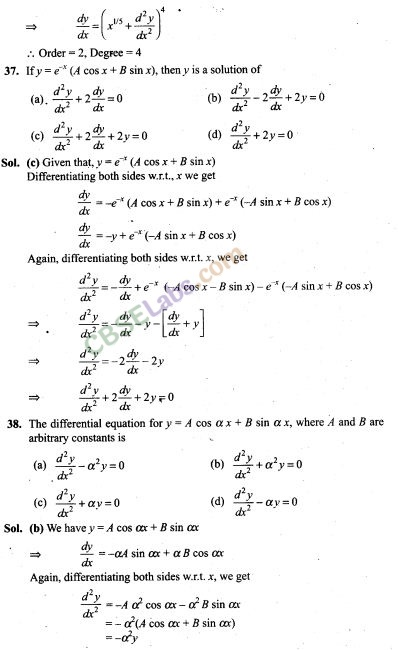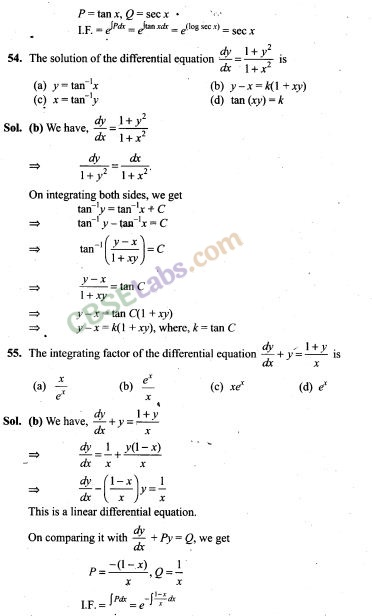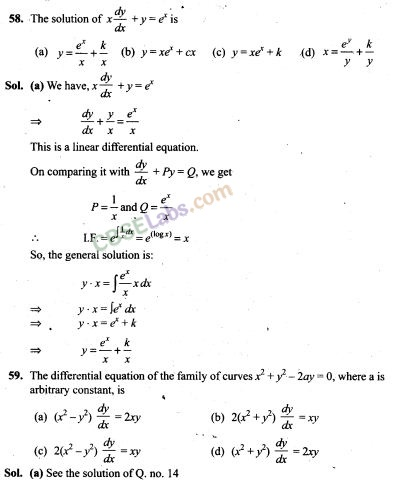60. Family y = Ax + A3 of curves will correspond to a differential equation of order ,
(a) 3 (b) 2 (c) 1 (d) not defined62. The curve for which the slope of the tangent at any point is equal to the ratio of the abscissa to the ordinate of the point is
(a) an ellipse (b) parabola
(c) circle (d) rectangular hyperbola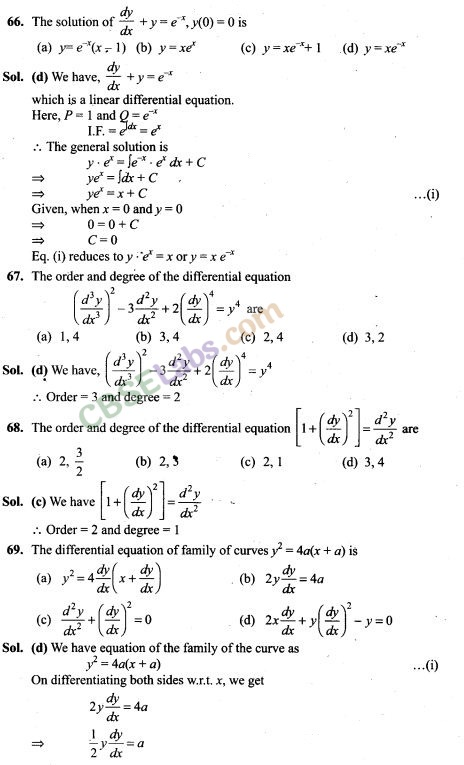Fill in the Blanks Type Questions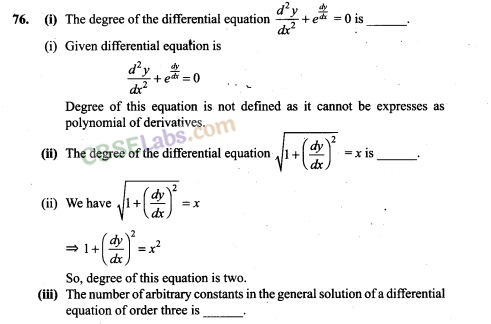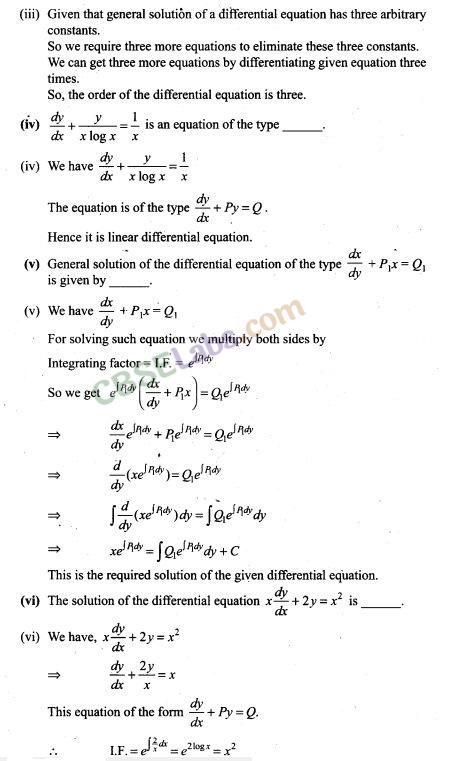True/False Type Questions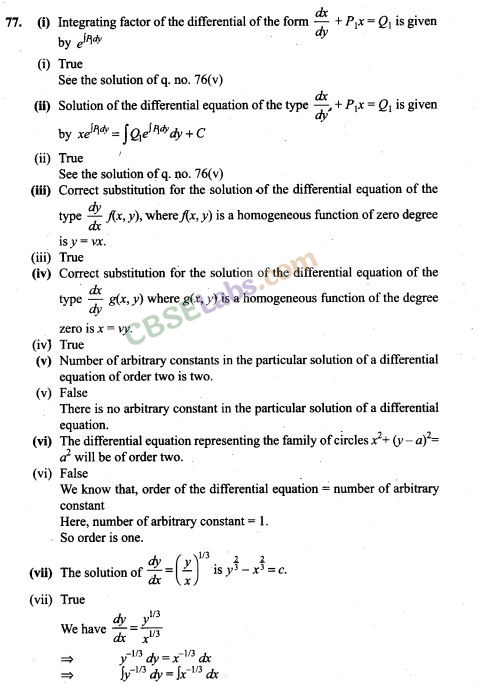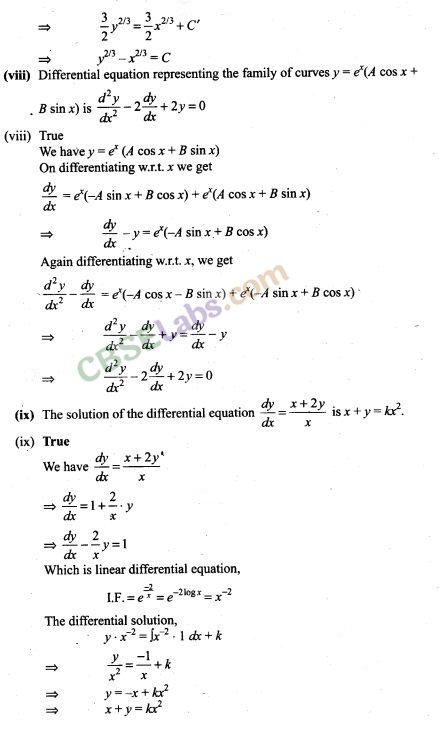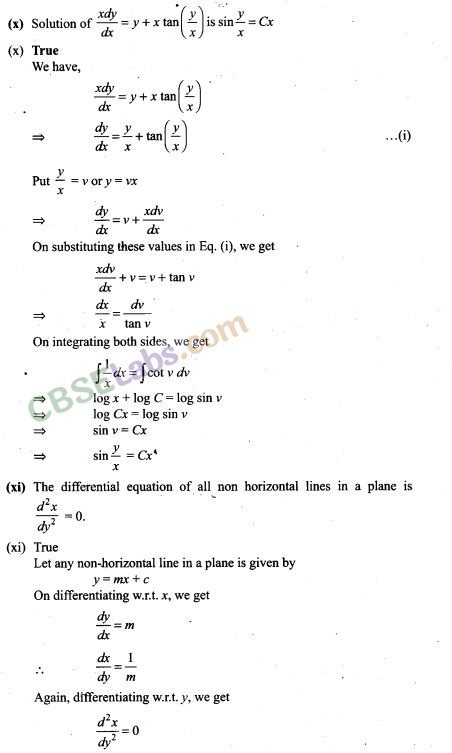## NCERT Exemplar Class 12 Maths Solutions

More Resources for Class 12 Maths

We hope the NCERT Exemplar Class 12 Maths Chapter 9 Differential Equations help you. If you have any query regarding NCERT Exemplar Class 12 Maths Chapter 9 Differential Equations, drop a comment below and we will get back to you at the earliest.